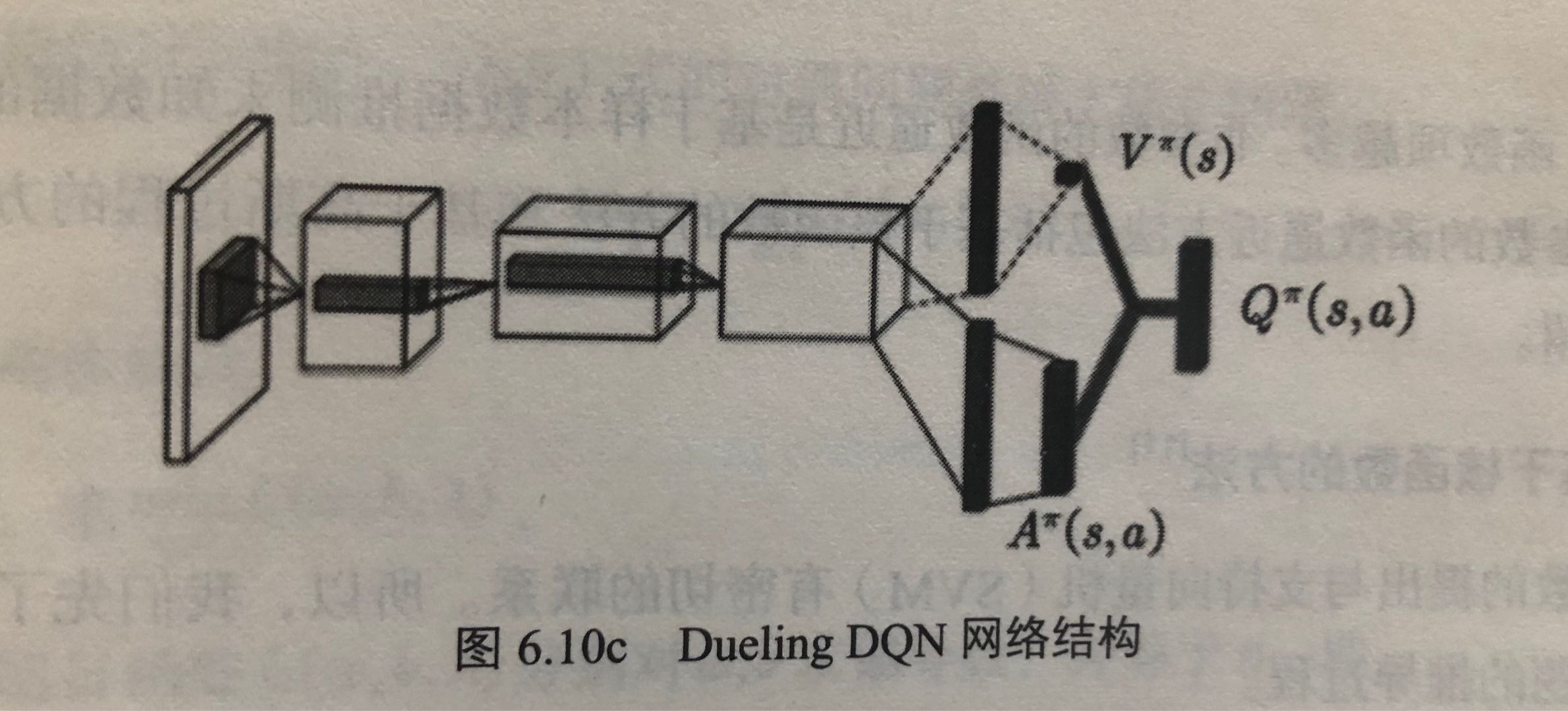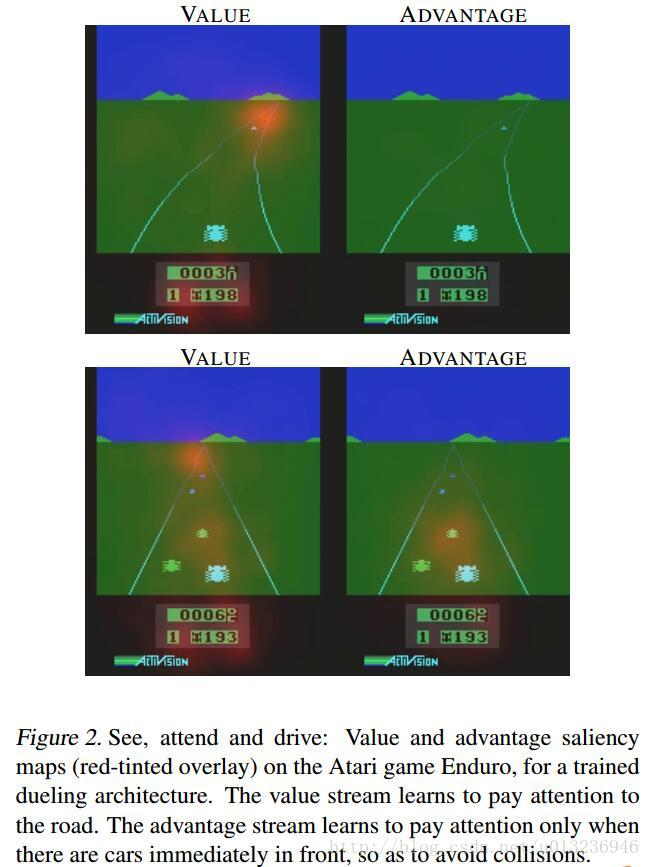To Top

## 2. DQN及其变种

### 2.1 DQN方法

DeepMind发表在Nature上的文章Human-level control through deep reinforcement learning

• 经验回放
• 设立单独的目标网络

• 异策略：行动策略要评估的策略不是同一个策略。
• 行动策略（用来选择行动$$a$$的策略）是$$\epsilon -greedy$$策略
• 要评估和改进的策略是贪婪策略（即$$max_aQ(s_{t+1},a)$$，当前状态$$s_{t+1}$$下，使用各种a使$$Q(s_{t+1},a)$$达到的最大值），即，是最大的$$Q$$，与行动策略里随机选一个$$a$$得到的$$Q$$不同
• 时间差分（temporal difference, TD）：使用时间差分目标（即，$$r_t+\gamma max_aQ(s_{t+1},a)$$）来更新当前的行为值函数

• 异策略(即off-policy)，如Qlearning，
• 同策略如Sarsa强化学习算法 （行为策略和目标策略都是$$\epsilon$$ -greedy策略）(书p77-79)

Qlearning的算法流程如下：

1. 初始化$$Q(s,a),\forall s\in S,a\in A(s)$$，给定参数$$\alpha, \gamma$$
2. Repeat
1. 给定起始状态$$s$$，并根据$$\epsilon -greedy$$策略在状态$$s$$选择动作$$a$$
2. Repeat
1. 根据$$\epsilon -greedy$$策略选择动作$$a_t$$，得到回报$$r_t$$和下一个状态$$s_{t+1}$$
2. 使用时间差分方法更新行为值函数$$Q(s_t,a_t)\leftarrow Q(s_t,a_t)+\alpha [r_t+\gamma max_a(Q(s_{t+1},a))-Q(s_t,a_t)]$$
3. $$s=s'$$, $$a=a'$$
3. Until $$s$$是最终状态
3. Until 所有的$$Q(s,a)$$收敛
4. 输出最终策略：$$\pi (s)=argmax_aQ(s,a)$$

DQN对Qlearning主要进行了如下三个修改：

• 利用深度卷积神经网络逼近行为值函数
• 在强化学习的学习过程中引入经验回放
• 设立了独立的目标网络单独处理时间差分算法中的TD偏差

#### 2.1.2 在强化学习的学习过程中引入经验回放

deepmind的创始人Hassabis是神经科学的博士，他是研究人类大脑中负责记忆和学习的主要部分——海马体的。在人类睡觉时，海马体会把一天的记忆重放给大脑皮层。

#### 2.1.3 设立了独立的目标网络单独处理时间差分算法中的TD偏差

$Q(s_t,a_t)\leftarrow Q(s_t,a_t)+\alpha [r_t+\gamma max_a(Q(s_{t+1},a))-Q(s_t,a_t)]$

$\theta_{t+1}=\theta _t+\alpha [r+\gamma max_{a'}(Q(s',a';\theta))-Q(s',a';\theta)]\nabla Q(s,a;\theta)$

$\theta_{t+1}=\theta _t+\alpha [r+\gamma max_{a'}(Q(s',a';\theta ^{-}))-Q(s,a;\theta)]\nabla Q(s,a;\theta)$

DQN的训练算法如下：

1. 初始化replay memory $$D$$，capacity设为$$N$$
2. 使用随机权重$$\theta$$初始化动作值函数$$Q$$
3. 使用权重$$\theta^{-}=\theta$$初始化目标动作值函数$$\hat {Q}$$
4. For $$episode = [1,...,M]$$ do
1. 初始化事件的第一个状态($$x_1$$是第一张图片)$$s_1=\{x_1\}$$，并通过预处理得到状态对应的特征输入$$\phi _1=\phi(s_1)$$
2. For $$t=[1,...T]$$ do
1. 根据概率$$\epsilon$$随机选择一个动作$$a_t$$
2. 如果小概率事件没有发生，就用贪婪策略选择当前行为值函数最大的那个动作：$$a_t=argmax_a(Q(\phi(s_t),a;\theta))$$【上面那行和这行就是所谓的行动策略，$$\epsilon -greedy$$策略】
3. 在模拟器中执行动作$$a_t$$，得到回报$$r_t$$以及图片$$x_{t+1}$$
4. $$s_{t+1}=s_t,a_t,x_{t+1}$$，然后预处理$$\phi _{t+1}=\phi(s_{t+1})$$
5. 将transition $$(\phi_t,a_t,r_t,\phi _{t+1})$$存入$$D$$
6. $$D$$中随机sample出一个minibatch的transitions，$$(\phi _j,a_j,r_j,\phi _{j+1})$$
7. $$y_j=\left\{\begin{matrix}r_j & if\ episode\ terminates\ at\ step\ j+1\\ r_j+\gamma max_{a'}\hat {Q}(\phi_{j+1},a';\theta^{-}) & otherwise \end{matrix}\right.$$
8. $$(y_j-Q(\phi _j,a_j;\theta))^2$$的参数$$\theta$$进行一个梯度下降step的更新，$$\theta_{t+1}=\theta _t+\alpha [r+\gamma max_{a'}(\hat {Q}(s',a';\theta ^{-}))-Q(s,a;\theta)]\nabla Q(s,a;\theta)$$
9. $$C$$个step，令$$\hat {Q}=Q$$，即令$$\theta ^{-}=\theta$$
3. End For
5. End For

#### DQN小结（by daiwk）

• $$y_i$$的时候用的是目标网络$$\hat{Q}$$$$\theta ^-$$
• loss是目标网络算出来的$$r+\gamma max \hat{Q}$$与原网络$$Q$$之差
• $$y_i$$是当前reward，基于状态$$s_{t+1}$$再执行一个action得到的新的reward。而原网络$$Q$$是当前的状态$$s_t$$
• 总共有这么几大步：
• 当前状态$$s_t$$，处理后是$$\phi _t$$，通过$$\epsilon -greedy$$得到$$Q$$最大的$$a_t$$
• 执行$$a_t$$，得到$$\phi _t, a_t,r_t,\phi_{t+1}$$，扔进replay buffer中
• 采样出一个$$\phi _j,a_j,r_j,\phi_{j+1}$$
• 计算$$\phi _{j+1}$$再执行一个使得$$\hat {Q}$$(注意，这里不是$$Q$$)最大的动作$$a'$$得到的reward：$$y_j=r_j+\gamma max \hat {Q}(\phi_{j+1},a')$$
• $$y_i$$和当前$$Q$$的残差，梯度下降，更新当前$$Q$$的参数：$$\theta += \alpha (y_j-Q)\nabla Q$$
• $$C$$步之后，把当前$$Q$$的参数赋值给$$\hat {Q}$$
• 再次强调
• $$maxQ$$的是当前状态+当前动作；
• $$max\hat {Q}$$下一状态+下一动作；
• 用来算残差的也是当前状态+当前动作得到的$$Q$$

### 2.2 Double DQN

#### 2.2.1 Qlearning的过估计

DQN无法克服Qlearning本身固有的缺点：过估计

$Q(s_t,a_t)\leftarrow Q(s_t,a_t)+\alpha [r_t+\gamma \underset{a}{max}Q(s_{t+1},a)-Q(s_t,a_t)]$

$\theta _{t+1}=\theta_t+\alpha(R_{t+1}+\gamma \underset{a}{max}Q(S_{t+1},a;\theta_t)-Q(S_t,A_t;\theta_t))\triangledown _{\theta_t}Q(S_t,A_t;\theta_t)$

$Y^Q_t=R_{t+1}+\gamma max_aQ(S_{t+1},a;\theta_t)$

• 动作选择

• 动作评估

Double Qlearning将动作的选择和动作的评估分别用不同的值函数来实现，从而其TD目标为：

$Y^{DoubleQ}_t=R_{t+1}+\gamma Q(S_{t+1},argmax_aQ(S_{t+1},a;\theta_t);\theta_t')$

$a^*=argmax_aQ(S_{t+1},s;\theta_t)$

$Y^{DoubleQ}_t=R_{t+1}+\gamma Q(S_{t+1},a^*;\theta_t')$

Deep Reinforcement Learning with Double Q-learning

$r+\gamma Q(s',argmax_{a'}Q(s',a',\theta),\theta^-)-Q(s,a,\theta)$

• $$\theta$$是当前的网络参数，用来选择动作
• $$\theta^-$$是前一步的网络参数（$$delayed \theta$$），用来评估动作

### 2.4 Dueling DQN

$Q^{\pi}(s,a)=V^{\pi}(s)+A^{\pi}(s,a)$

• 状态值函数$$V^{\pi}(s)$$表示静态的状态环境本身具有的价值。是一个scalar。
• 依赖状态的动作优势函数$$A^{\pi}(s,a)$$(advantage function)，表示选择某个Action额外带来的价值。是一个vector。

$V(s;\theta,\beta)$

$A(s,a;\theta,\alpha)$

$Q(s,a;\theta,\alpha,\beta) = V(s;\theta,\beta)+A(s,a;\theta,\alpha)$

$Q(s,a;\theta,\alpha,\beta) = V(s;\theta,\beta)+(A(s,a;\theta,\alpha)-\frac{1}{|A|}\sum_{a'}A(s,a';\theta,\alpha))$## 4. 各种其他优化

• 目标Q值的计算准确吗？全部通过max Q来计算有没有问题？
• 随机采样的方法好吗？按道理不同样本的重要性是不一样的
• Q值代表状态，动作的价值，那么单独动作价值的评估会不会更准确？
• DQN中使用$$\epsilon-greedy$$的方法来探索状态空间，有没有更好的做法？
• 使用卷积神经网络的结构是否有局限？加入RNN呢？
• DQN无法解决一些高难度的Atari游戏比如Montezuma’s Revenge，如何处理这些游戏？
• DQN训练时间太慢了，跑一个游戏要好几天，有没有办法更快？
• DQN训练是单独的，也就是一个游戏弄一个网络进行训练，有没有办法弄一个网络同时掌握多个游戏，或者训练某一个游戏后将知识迁移到新的游戏？
• DQN能否用在连续动作输出问题？

comment here..